# N-way Unequal-Split Wilkinsons

This page was contributed by Paul Hubbard and Greg Ordy of Seed Solutions, originally in August 2009. During August 2011 it was peer reviewed by John, and we posted the missing equation graphics (finally). Thanks, guys! Here the design equations are derived that determine the parameters for arbitrary power splits.

### Introduction

While designing a circuit to cancel noise in an amateur radio project, we needed a 3-way Wilkinson divider/combiner with unequal power split: 80% on one arm and 10% on each of the other two. We couldn't find any design equations for such a thing on the web or in our favorite book , but it seemed like such a thing must be possible. The folks at microwaves101.com even had some new equations  that suggested there was more gold to be mined in the world of unequal split Wilkinsons. Against this backdrop we sharpened our pencils and developed a set of equations to assist in the design of an N-way unequal split Wilkinson divider, using a star configuration for the isolation resistors.

We understand why these design equations aren't widely available: it's hard to implement N-way Wilkinsons, and the impedance ratios required to split power unequally can quickly get out of hand. For our low frequency application, we implement the quarter wavelength transmission lines using lumped elements, so many of the constraints seen by microstrip designers don't apply to us. We present these design equations for completeness, and in the hope that the Wilkinson power divider can be better understood by more designers.

### Design Equations

Figure 1 shows an annotated schematic for a generalized N-way unequal Wilkinson Divider. Each of the boxes labeled Zk (which is shorthand notation in this paper for one of ZA, ZB, â€¦ ZN) is a quarter wavelength ( /4) transmission line, with characteristic impedance Zk. The isolation resistors use the "star" configuration. The solid line blue, dashed line green and dotted line red arrows show the impedances which must be seen looking into each arm at that point in the circuit. The only difference between this diagram and an equal N-way Wilkinson divider is that all of the impedances have unique names, since they can all be different for an unequal split.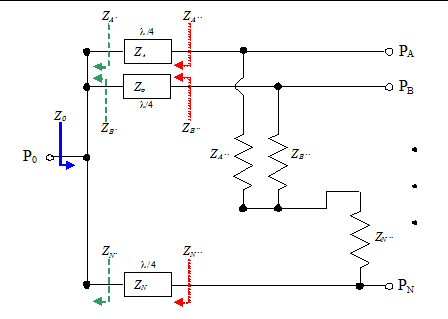Figure 1. N-way Unequal Wilkinson Divider

The goal of this paper is to describe the design equations that constrain the values for the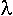/4 transmission line impedances and isolation resistors. We will see that the designer gets to choose the impedance seen looking into the input port (Z0), the desired power split, and the impedance seen looking into one of the output ports (some Zk''). All of the other impedances in the circuit can then be computed.

Starting at the left side of Figure 1, the input, the designer chooses the impedance seen looking into P0 (the solid line blue arrow). Call it Z0.

Next, the designer must choose how power is divided among the arms of the circuit. We choose to express the division as a percentage of power present at the input delivered into each arm. Because all of the arms are tied together at the input, the voltage present at the input side of each arm is identical. Thus, the power delivered to each arm is proportional to the current flowing down each arm. So, the desired power division can be specified as the sum of the currents flowing down each arm, summing to a mythical 1 Amp: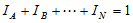(0<IK<1 ) (1a)

These currents must be coaxed (as in persuaded, not the cable!) down each arm of the divider by having each arm present the appropriate impedance, which we call Zk' (the dashed line green arrows in Figure 1). Given that the currents sum to 1 Amp, and that 1 Amp is being delivered into a load of Z0 Ohms, we must have Z0 Volts present at the input. Since I=V/R (Ohm's Law), it must be true that: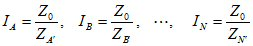(1b)

Or, by rearranging: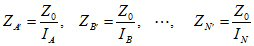(1c)

It is easy to see, given (1a) and (1c), that:(1d)

Equation (1d) is one of the equations that must be satisfied for proper Wilkinson operation: the impedances of the arms must combine in parallel to provide the impedance Z0 .

We now turn to the impedances of each of the/4 transmission lines. One of the many interesting properties of a/4 transmission line is that the voltage at one end of the line is the product of the current at the other end of the line and the characteristic impedance of the line: VFar=INear ZCharacteristic. This is the so-called "current forcing" property. For proper Wilkinson operation, there must be no current flowing in any of the isolation resistors when power is applied to P0. In order for this to be true, the voltage at the output side of each /4 transmission line must be the same when power is applied to P0. Using this fact and current forcing, we have the following system of N-1 equations in N unknowns: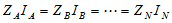(2)

Equation (2) establishes ratios of /4 transmission line characteristic impedances between each pair of arms in the divider, since we know all of the Ik values (they were selected by the designer, subject to equation (1a)), but none of the Zk values (yet!).

Equation (2) emphasizes a point worth repeating: the voltage seen at each output, Pk, is the same when power is applied to P0. This is not intuitive in the context of an unequal power split. The power split is achieved because that voltage is delivered into different loads. The impedance of the loads is thus inversely proportional to the power delivered to each arm.

Next, we consider the impedance, Zk'', seen when looking into the output of each arm of the divider (the dotted line red arrows in Figure 1). Each/4 transmission line is an impedance transformer, acting on Zk' to produce Zk'' via its characteristic impedance, Zk, according to: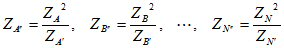(3)

Finally, we turn to the isolation resistor values. It has been shown elsewhere that the isolation resistor values for a star configuration N-way divider are equal to the Zk'' values for each arm of the divider, and the same is true here. So far, we have only been considering an excitation mode where there is no current flow in the isolation resistors (sort of an even mode analysis). Other Wilkinson divider analyses use an odd mode excitation, along with symmetry arguments, to show what the isolation resistor values must be when all of the power incident at the output ports is dissipated in the resistors and none makes it to the input port (P0). A similar argument applies here: when the outputs are driven in such a way that all power should be dissipated in the isolation resistors, each resistor must present the same impedance as its arm presented in the even mode case.

We now have all of the equations we need, but we still need one more piece of information from the designer: one of the Zk'' impedance values must be chosen. Once that choice has been made, we can solve for Zk using the appropriate clause of equation (3). With that Zk value, we can solve for the remaining Zk using equation (2). From there, equation (3) is used to calculate the remaining Zk''.

### Conclusions

We have developed design equations for an N-way unequal split star configuration Wilkinson divider. The designer supplies an input impedance (Z0), the division of power among the arms of the divider (expressed as a sum of currents flowing down the arms), and the impedance seen looking back into one of the outputs of the divider. With this information, the characteristic impedance of each/4 transmission line and the value of each isolation resistor can be computed.

Equation (2) emphasizes a characteristic not usually seen in Wilkinson analyses: the voltage seen at each output, Pk, is the same when the divider is driven from P0, independent of power split across the arms.

We left the equations in a form that forces the designer to do some "plugging and chugging" in the hope that the intuition behind the equations would be clearer. More insight can be gained from further manipulation of the equations. For example:

• The design equations for the classic 2-way, N-way star configuration, and unequal split Wilkinson are consistent with the equations presented here. We say "consistent with" because the classic design equations impose additional impedance constraints that are not strictly required for proper Wilkinson operation.
• The design equations presented in  are derivable from the equations presented here, if you modify the circuit shown here to include the output transformers. Both sets of equations allow the same number of degrees of freedom for the 2-way unequal case.
• The impedances are inversely proportional to the current flowing through the corresponding arm of the divider.
• The impedance ratios across arms remain constant. That is: Zj'/Zk' = Zj/Zk = Zj''/Zk'' for all j and k.
The equal split Wilkinsons (2-way and N-way star configuration) do not need to have the same input and output impedances (though all the outputs must have the same impedance).
• We have presented the equations from a perspective that implies the designer can not directly choose the characteristic impedance of any/4 transmission line. This is not the case: one could choose a/4 transmission line impedance if one were willing to give up the choice of either Z0 or Zk''.

### References

 David M. Pozar, Microwave Engineering, Third Edition, Section 7.3 "The Wilkinson Power Divider", John Wiley & Sons: New York, 2005.

 "Unequal-Split Wilkinsons - the Rest of the Story - Microwave Encyclopedia - Microwaves101.com", https://www.microwaves101.com/encyclopedias/unequal-split-wilkinsons-the-rest-of-the-story. Retrieved on August 4, 2009.

### Example 1: 2-way with different input and output impedances

Consider a standard 2-way Wilkinson with an added wrinkle: the input impedance, Z0, is 75 Ohms while the output impedances are 50 Ohms. So, our givens are:

Z0=75
IA+IB=0.5+0.5=1
ZA''=50

From equation (1c), we have:

ZA'=ZB'=75/0.5=150

From the first clause of equation (3), we get:

50=ZA2/150 or ZA=86.6

And, by symmetry:

ZB''=50 and ZB=86.6

Here's some plots that back up the analyses... UE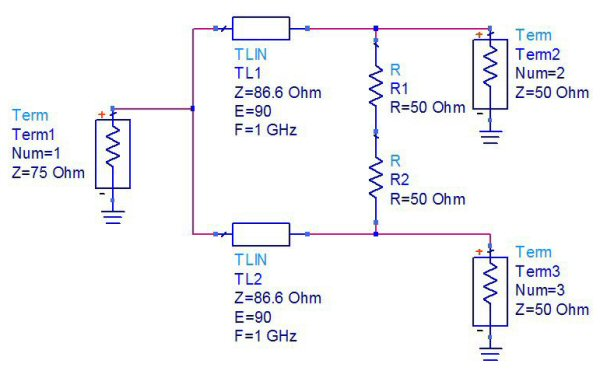Example 1 schematic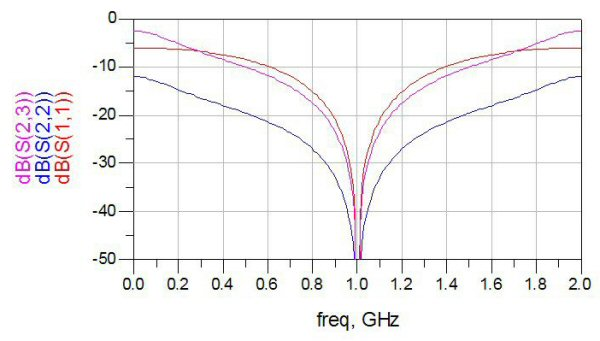Example 1 return losses and isolation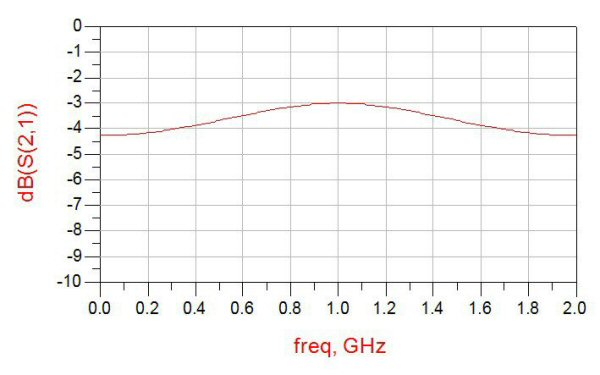Example 1 transmission

### Example 2: 3-way, 80/10/10 split

Let's consider the example that caused us to develop these equations: a 3-way Wilkinson with 80/10/10 power split. For our application, we need the 80% port to be 50 Ohms.

The value of Z0 does not really matter in our application, and we choose it to be 25 Ohms. Why 25 Ohms? We know from equation (2) that we will experience impedance ratios in the /4 transmission lines of 8:1. By choosing Z0=25, the impedances of these lines are easier to realize than if we were to choose a higher value for Z0. Here is a case where understanding where we are constrained and where we have choices allows us to make a more informed design decision.

Thus, we are given:

Z0=25
IA+IB+IC=0.1+0.1+0.8=1
ZC''=50

From equation (1c), we get:

ZC'=25/0.8=31.25
ZA'=ZB'=25/0.1=250

From the third clause of equation (3), we have:

50=ZC2/31.25 or ZC=39.52

Using equation (2), we get:

ZC IC=39.52 0.8=31.62
ZA IA=ZB IB=31.62 or ZA=ZB=31.62/0.1=316.2

And, from equation (3), we have:

ZA''=ZB''=316.22/250=400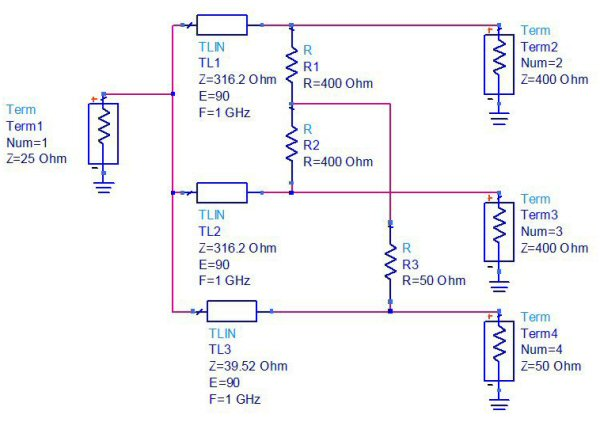Example 2 schematic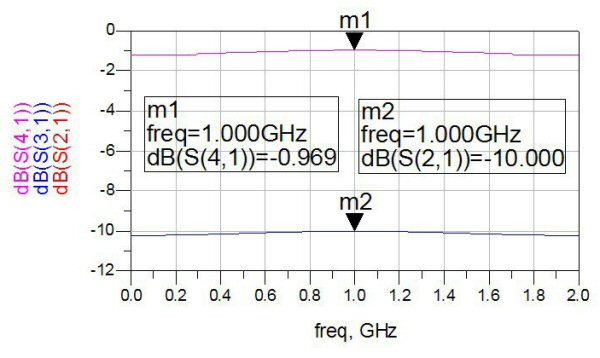Example 2 transmission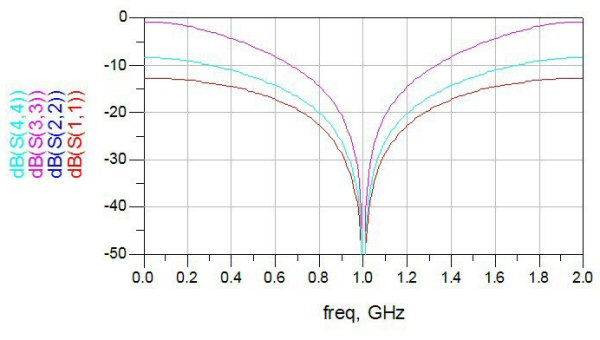Example 2 return losses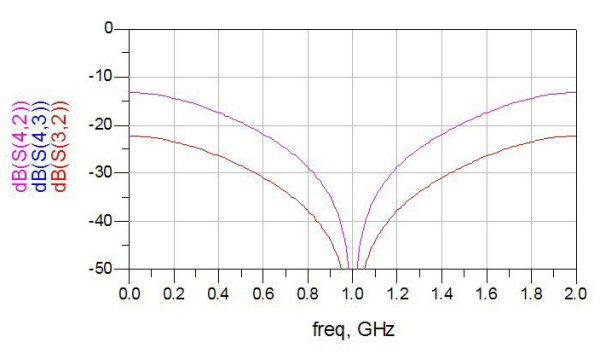Example 2 isolations

Microwaves101  reproduces parts of Split Tee Power Divider, by Parad and Moynihan, published in IEEE Transactions on Microwave Theory and Techniques in January, 1965. In particular, they reference the equations for the impedance seen looking into each output of the basic power divider: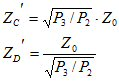The web page says these equations are "based on an assumption that is not fully explained in the paper", and solicits an explanation.

We haven't read the Parad and Moynihan paper, so we won't presume to explain their assumptions. However, the equations presented in this paper allow us to see where the authors were constrained, and where they were making their own design decisions. Perhaps this will help others arrive at the explanation sought.

We know from equation (2) in this paper that the ratio of the output arm impedances is set by the power division. This can be verified by taking the ratio of the Parad and Moynihan equations: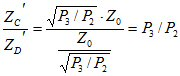So, if we could ask two questions to help explain the Parad and Moynihan equations, they would be:

1. Why was the ratio of output impedances (P3/P2) expressed as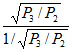?

2. Why were the numerator and denominator of this ratio then scaled by Z0 to arrive at the final output impedance values?

The equations in this paper (and the analysis done in ) show that any impedance could have been chosen for one of the outputs and the impedance of the other output could have then been computed.

If forced to speculate, we would guess that Parad and Moynihan wished to express both of the output impedances as Z0 scaled by a constant (as is often done in the Wilkinson literature), coupled with a desire to minimize the composite excursion from Z0 on each arm of the divider measured as a ratio with respect to Z0, and that these decisions then led to the equations in question.

For a 2-way, unequal split Wilkinson, we can postulate an impedance ratio presented by the arms: b/a=k. k is the actual ratio, and b and a are the scale factors applied to Z0 to produce the actual impedances seen looking into the arms of the divider. The desire is to simultaneously minimize b/Z0 and Z0/a. We express this thusly: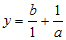This equation takes the perspective that k > 1, b > 1, and a < 1. The ones represent Z0 normalized out of the ratios. Substituting kA for b, we get: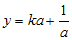Taking the derivative with respect to a, and equating to 0, we get: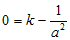Solving for a: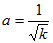Solving for b: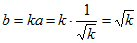So, assuming that Parad and Moynihan's goal was to minimize the ratiometric excursion from Z0 in the intermediate arm impedances, we see why they chose P4/P5=1/K2, and we get answers to our 2 questions above.

### Postscript: Minimum Excursion From Z0

While working on the previous addendum, we thought that perhaps Parad and Moynihan had chosen the intermediate impedances because they possessed some useful property, like a minimum total excursion from Z0. This turned out not to be the case, but the exercise was useful, so here it isâ€¦

For a 2-way, unequal split Wilkinson, we postulate an impedance ratio presented by the arms: b/a=k. k is the actual ratio, and b and a are the scale factors applied to Z0 to produce the actual impedances seen looking into the arms of the divider. The desire is to simultaneously minimize the distance from b and a to 1. We express this using least squares: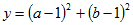Or, since b=kA: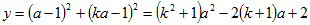Taking the derivative and equating to 0, we get: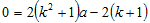And then: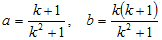(4)

Equation (4) gives the scale factors that represent the minimum overall excursion from Z0, measured in Ohms, for each arm of the divider.

As an example, let's look at a power split of 4:1. Using the Parad and Moynihan equations: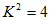And the intermediate impedances for a 50 Ohm system would be: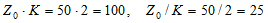Using equation (4), k=4, the intermediate impedances for a 50 Ohm system would be: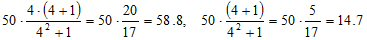Author : Paul Hubbard and Greg Ordy